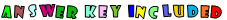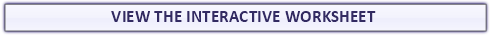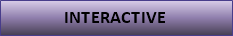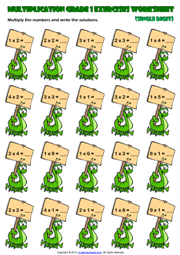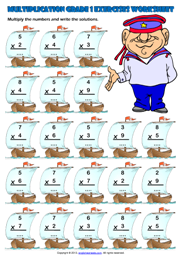# Grade 1 Multiplication Printable Maths Worksheets and Exercises

Here on this page you can download and use Printable Grade 1 Maths Worksheets, Exercises, Handouts, Tests, Activities, Teaching and Learning Resources, Materials  for kids about Multiplication

## Single Digit Multiplication Maths Exercise Worksheet

A fun multiplication exercises maths worksheet for grade 1 (first grade) students and kids with ships and sailor theme. Multiply the numbers and write the solutions. (Vertical Multiplication Worksheet for kids)

## First Grade Single Digit Multiplication Exercise Worksheet

An easy multiplication exercise maths worksheet for grade 1 (first grade) students and kids with caterpillars theme. Multiply the numbers and write the solutions. Single Digit Multiplicants, Multipliers and results.
(Horizontal
Multiplication Worksheet for kids)